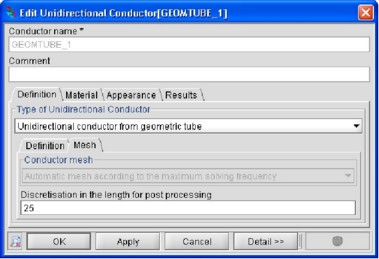## Plotting of arrows

Spatial vectorial quantities can be displayed by means of colored arrows on the different supports.

## Support

The support for the plotting of arrows are:

• unidirectional conductors
• bidirectional conductors
• 2D grids

## Spatial quantities

The spatial quantities that can be displayed by means of arrows are:

• the current density and its three components (X, Y or Z)
• the magnetic flux density and its three components (X, Y or Z)
• the Laplace force density (the average component as well as the pulsating component) and its three components (X, Y or Z)

## Meaning of the arrows

The spatial quantities considered are displayed by means of arrows according to the following rules:

• the color of the arrows is proportional to the modulus of the quantity
• the orientation of the arrows points the direction of this quantity*
Note: * Obviously, if the quantity selected is a component of a quantity, the orientation of the arrows will be the direction of that component.

## Computation points

The points where the arrows are computed depends on the spatial quantity selected but are in general highly linked to:

• for unidirectional conductors, to the mesh and the additional points due to the discretization in the length for the post-processing*
• for bidirectional conductors, to the mesh
• for 2D grids, to their own discretization as described in § 2D grids: about
Note: * To discretize in the length a unidirectional conductor, the user must first create it and then edit its features from the Data tree. In the Mesh tab of the window which appears, the user can define the number of discretization points along the length, as shown in the figure below.